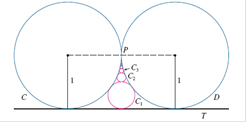### Create an Account

Home / Questions / The figure shows two circles C and D of radius 1 that touch at P. T is a common

# The figure shows two circles C and D of radius 1 that touch at P. T is a common

The figure shows two circles C and D of radius 1 that touch at P. T is a common tangent line; C1 is the circle that touches CD, and TC2 is the circle that touches CD, and; is the circle that touches CD, and C2. This procedure can be continued indefinitely and produces an infinite sequence of circles {Cn}. Find an expression for the diameter of { Cn} and thus provide another geometric demonstration of Example 6.EXAMPLE 6 Show that the seriesis convergent, and find its sum.

Apr 24 2020 View more View LessSubscribe To Get Solution